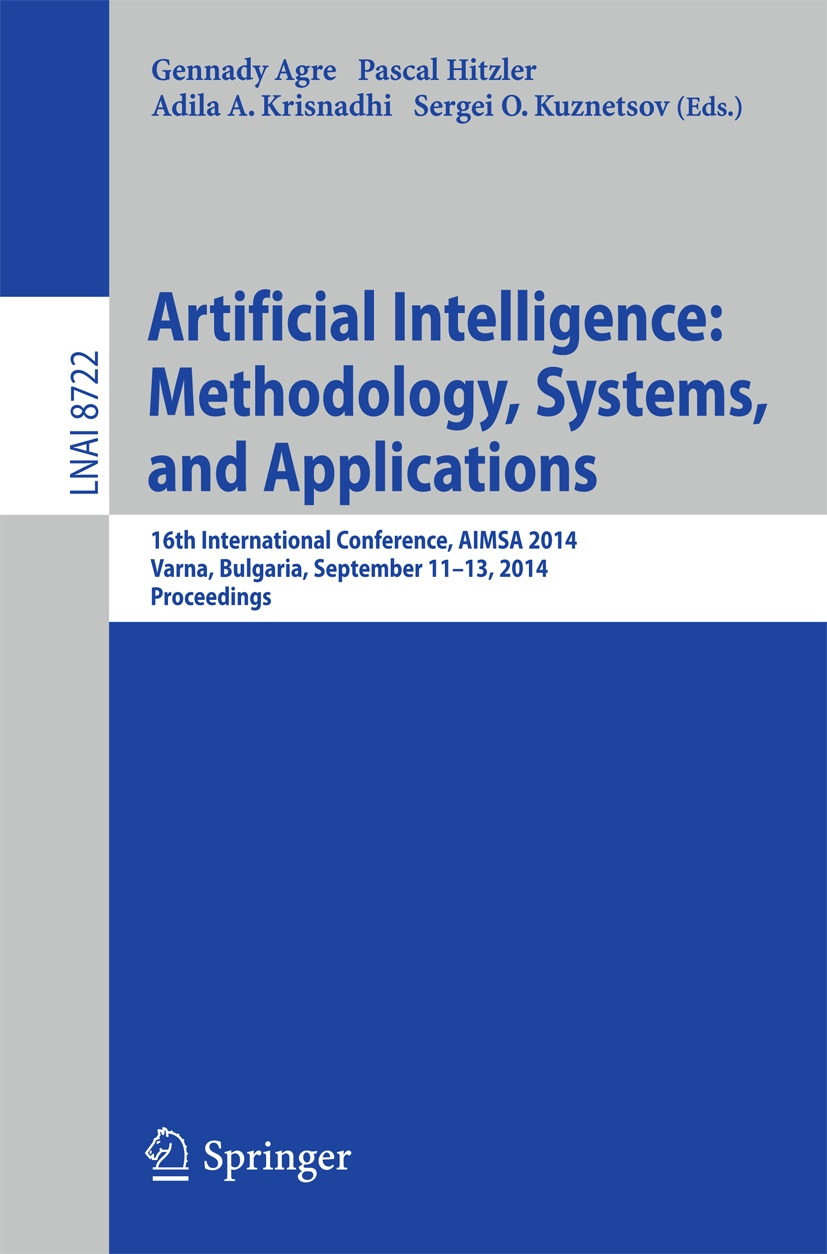• A
• A
• A
• ABC
• ABC
• ABC
• А
• А
• А
• А
• А
Regular version of the site

## Feature Selection by Distributions Contrasting

P. 139-149.
Varvara V. Tsurko, Michalski A. I.

We consider the problem of selection the set of features that are the most significant for partitioning two given data sets. The criterion for selection which is to be maximized is the symmetric information distance between distributions of the features subset in the two classes. These distributions are estimated using Bayesian approach for uniform priors, the symmetric information distance is given by the lower estimate for corresponding average risk functional using Rademacher penalty and inequalities from the empirical processes theory. The approach was applied to a real example for selection a set of manufacture process parameters to predict one of two states of the process. It was found that only 2 parameters from 10 were enough to recognize the true state of the process with error level 8%. The set of parameters was found on the base of 550 independent observations in training sample. Performance of the approach was evaluated using 270 independent observations in test sample.

### In bookEdited by: G. Agre, P. Hitzler, A. A. Krisnadhi et al. Vol. 8722. Cham; Heidelberg; NY; Dordrecht; L.: Springer, 2014.